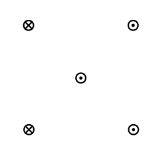# Problem: As shown in the figure, four long parallel wires lie on the corners of a square, with a 5th at the center. All wires carry identical currents, with directions shown in the figure (a cross inside a circle means the current is into the page while a dot inside a circle means the current is out of the page). What is the direction of the net force on the central wire (angles are measured from the +x axis)? (1) 0° (2) 45° (3) 90° (4) 135° (5) 270°

###### FREE Expert Solution
93% (136 ratings)
###### Problem Details

As shown in the figure, four long parallel wires lie on the corners of a square, with a 5th at the center. All wires carry identical currents, with directions shown in the figure (a cross inside a circle means the current is into the page while a dot inside a circle means the current is out of the page). What is the direction of the net force on the central wire (angles are measured from the +x axis)?

(1) 0°

(2) 45°

(3) 90°

(4) 135°

(5) 270°Frequently Asked Questions

What scientific concept do you need to know in order to solve this problem?

Our tutors have indicated that to solve this problem you will need to apply the Magnetic Force Between Parallel Currents concept. You can view video lessons to learn Magnetic Force Between Parallel Currents. Or if you need more Magnetic Force Between Parallel Currents practice, you can also practice Magnetic Force Between Parallel Currents practice problems.

How long does this problem take to solve?

Our expert Physics tutor, Juan took 2 minutes and 56 seconds to solve this problem. You can follow their steps in the video explanation above.

What professor is this problem relevant for?

Based on our data, we think this problem is relevant for Professor Chen's class at SFU.